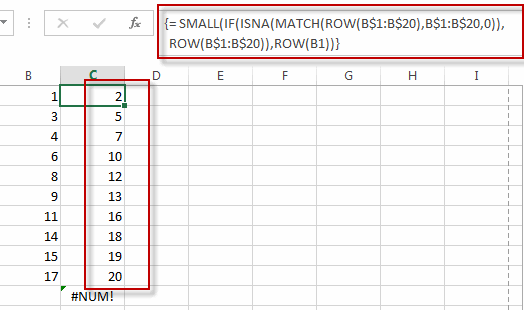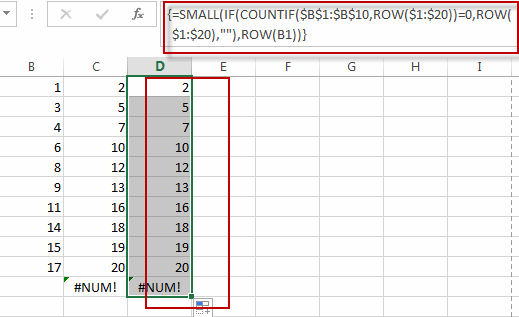# Find Missing Numbers in a Sequence in Excel

This post will guide you how to find missing numbers in a sequence with a formula in Excel. How do I identify missing numbers in a consecutive series in Excel. How to find missing serial number in Excel 2013/2016.

## Find Missing Numbers in a Sequence in Excel

Assuming that you have a serial number list in Column B, and you want to find the missing number in this sequence list. How to achieve it.

You can use an excel array formula based on the SMALL function, the IF function, the ISNA function, the MATCH function, and the ROW function. Like this:

=SMALL(IF(ISNA(MATCH(ROW(B\$1:B\$20),B\$1:B\$20,0)),ROW(B\$1:B\$20)),ROW(B1))

Type this array formula into a blank cell, and then press Ctrl + Shift + Enter keys in your keyboard.The missing numbers are listed in cells.

Note: this formula will check the given sequence from 1 to 20 if there are missing numbers. If so, returns the missing numbers.

Or you can use another array formula based on the Small function, the IF function, the Countif function and the row function to achieve the same result. Like this:

=SMALL(IF(COUNTIF(\$B\$1:\$B\$10,ROW(\$1:\$20))=0,ROW(\$1:\$20),””),ROW(B1))### Related Functions

• Excel MATCH function
The Excel MATCH function search a value in an array and returns the position of that item.The syntax of the MATCH function is as below:= MATCH  (lookup_value, lookup_array, [match_type])….
• Excel SMALL function
The Excel SMALL function returns the smallest numeric value from the numbers that you provided. Or returns the smallest value in the array.The syntax of the SMALL function is as below:=SMALL(array,nth) …
• Excel ROW function
The Excel ROW function returns the row number of a cell reference.The ROW function is a build-in function in Microsoft Excel and it is categorized as a Lookup and Reference Function.The syntax of the ROW function is as below:= ROW ([reference])….
• Excel IF function
The Excel IF function perform a logical test to return one value if the condition is TRUE and return another value if the condition is FALSE. The IF function is a build-in function in Microsoft Excel and it is categorized as a Logical Function.The syntax of the IF function is as below:= IF (condition, [true_value], [false_value])….
• Excel COUNTIF function
The Excel COUNTIF function will count the number of cells in a range that meet a given criteria. This function can be used to count the different kinds of cells with number, date, text values, blank, non-blanks, or containing specific characters.etc.= COUNTIF (range, criteria)…

Related Posts

Excel Array Construction

This article will talk about how to create one-dimensional array or two-dimensional array by using some functions in Excel. When using array formulas in Excel, we often use functions to construct arrays. Generate Array with ROW or COLUMN Functions Array ...

Excel Array

What is Excel Array? In Excel functions and formulas, an array is a collection of data elements in one row, one column, or multiple rows and columns. Array elements can be numeric, text, date, logical and error values. The dimension ...

Extract matching values From Two Lists

Suppose that you are working with two lists containing few values, and you want to extract the matching values from those two lists into another separate list. You might prefer to manually extract the matching values from the two lists, ...

Extract all Partial Matches

Just assume that you have a range of data that contains a text string and you want to extract the few partial matches into another separate range of cells; then you might think that it's not a big deal; because ...

Extract Multiple Match Values into Separate Columns

If you have a few values/items in the excel sheet and you are thinking that with the aid of the “VlOOKUP” function you can look for a specific value, extract it and then put the matching item into the separate ...